Objects and Methods : Reservoir Methods : User Methods : Evaporation and Precipitation
Evaporation and Precipitation
The Evaporation and Precipitation category methods are used to calculate the volume of Evaporation from and Precipitation to the surface of a reservoir over the timestep. Precipitation and Evaporation are used in the mass balance equations which are solved in the dispatch methods.
Some of the methods in this category only calculate evaporation.None
The None method is the default method for the Evaporation and Precipitation category. It should be chosen if the user does not want to include Evaporation in the mass balance equation of the Reservoir. No calculations are performed by this method.
There are no slots specific to this method.Daily Evaporation
The Daily Evaporation method is used to calculate the daily evaporation volume and the flow rate of the precipitation. The daily evaporation volume is a function of the Evaporation Rate, average Surface Area, and Pan Coefficient.
Slots Specific to This Method
Elevation Area Table
Type: Table Slot
Units: LENGTH vs AREA
Description: Pool Elevation vs Surface Area
Information:
I/O: Required input
Evaporation
Type: SeriesSlot
Units: VOLUME
Description: Volume of water lost to evaporation during one timestep
Information:
I/O: Output only
Evaporation Table
Type: Table Slot
Units: NOUNITS vs LENGTH (per day)
Description: Day of the year vs Evaporation Rate
Information: The first of January is 0. The unit type of second column is Length, but this actually implies a rate (length/time) where the time component is the run timestep.
I/O: Required input
Pan Evaporation Coefficient
Type: Table Slot
Units: NO UNITS
Description: Fractional value between 0 and 1 that represents the portion of potential evaporation which actually occurs
Information:
I/O: Required input
Precipitation Rate
Type: SeriesSlot
Units: LENGTH vs TIME
Description: Precipitation intensity for the given timestep
Information:
I/O: Optional; defaults to 0.0 if not input.
Precipitation Volume
Type: SeriesSlot
Units: VOLUME
Description: Precipitation flow rate multiplied by the length of the timestep
Information: Used in the mass balance to solve for storage
I/O: Output only
Surface Area
Type: SeriesSlot
Units: AREA
Description: Reservoir Surface Area calculated from the Elevation Area Table
Information:
I/O: Output only
Method Details
The Surface Area is determined using the Pool Elevation and the Elevation Area Table. The Evaporation Rate is looked up in the Evaporation Table according to the current day of the year. Evaporation is calculated using the following equation: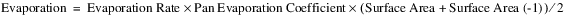The volume of Precipitation that occurred over the timestep is then calculated with the following equation: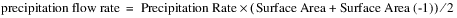where:
– Evaporation Rate = the Evaporation Rate corresponding to the current day of the year
– Surface Area = the current Surface Area of the Reservoir
– Surface Area(-1) = the Surface Area of the Reservoir at the previous timestepInput Evaporation
The Input Evaporation method should be used when the user wants to input the Evaporation Rate directly. This Evaporation Rate is used to compute the volume of water that evaporated over the timestep. Also, the user can input the evaporation volume directly on the Evaporation slot. In that case, the Evaporation Rate is not used to calculated Evaporation.
Slots Specific to This Method
Elevation Area Table
Type: Table Slot
Units: LENGTH vs AREA
Description: Pool Elevation vs Surface Area
Information:
I/O: Required input
Evaporation
Type: SeriesSlot
Units: VOLUME
Description: Volume of water lost due to evaporation during the timestep
Information:
I/O: Output; optional input overrides calculation
Evaporation Rate
Type: SeriesSlot
Units: LENGTH per TIME
Description: Rate at which water evaporates from the surface
Information:
I/O: Optional input, disaggregated by method as described in the Evap and Precip Rate Specification category, or defaults to 0.0 if not specified by the user.
Precipitation Rate
Type: SeriesSlot
Units: LENGTH per TIME
Description: Precipitation intensity for a given timestep
Information:
I/O: Optional input, disaggregated by method as described in the Evap and Precip Rate Specification category, or defaults to 0.0 if not specified by the user.
Precipitation Volume
Type: SeriesSlot
Units: VOLUME
Description: Precipitation flow rate multiplied by the length of the timestep
Information: Used in the mass balance to solve for storage
I/O: Output only
Surface Area
Type: SeriesSlot
Units: AREA
Description: Reservoir Surface Area from the Elevation Area Table
Information:
I/O: Output only
Method Details
At the beginning of the run, the chosen method in the Evap and Precip Rate Specification category is executed. This category allows you to specify the rates as monthly or periodic slots.
If the user specifies Evaporation directly (via input or rules), the value will be used instead of calculating a value below.
If Evaporation is not specified, the following equation is used to compute the volume of water that evaporated from the Reservoir over the timestep: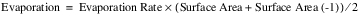The precipitation flow rate over the timestep is calculated as shown in the following equation: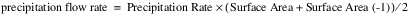where:
– Surface Area = the current Surface Area of the Reservoir
– Surface Area(-1) = the Surface Area of the Reservoir at the previous timestepMonthly Evaporation
In the Monthly Evaporation method, evaporation is calculated linearly from the Evaporation Coefficients entered for each month. This method will not work with a timestep longer than 1 Month. The total evaporated volume is a function of the average Reservoir Surface Area over the timestep, the Evaporation Coefficient, and the length of the timestep. The following slots are specifically associated with this method.
Slots Specific to This Method
Elevation Area Table
Type: TableSlot
Units: LENGTH vs AREA
Description: Pool Elevation vs Surface Area
Information:
I/O: Required input
Evaporation
Type: SeriesSlot
Units: VOLUME
Description: Volume of water lost to evaporation during one timestep
Information:
I/O: Output only
Evaporation Coefficients
Type: TableSlot
Units: LENGTH PER time
Description: Rate of evaporation for each month
Information: This slot contains one column of values. The Evaporation Coefficient for each month of the year must be input by the user beginning with the Evaporation Coefficient for January.
I/O: Required input
Precipitation Rate
Type: SeriesSlot
Units: LENGTH per TIME
Description: Precipitation intensity for the given timestep
Information: Value must be input by the user for each timestep.
I/O: Optional; defaults to 0.0 if not input.
Precipitation Volume
Type: SeriesSlot
Units: VOLUME
Description: Precipitation flow rate multiplied by the length of the timestep
Information: Used in the mass balance to solve for storage
I/O: Output only
Surface Area
Type: SeriesSlot
Units: AREA
Description: Reservoir Surface Area calculated from the Elevation Area Table
Information:
I/O: Output only
Method Details
The Surface Area of the Reservoir is calculated based on the Elevation Area Table. The Evaporation is then calculated using the following formula: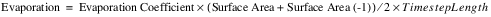The volume of Precipitation that occurred over the timestep is then calculated using the following equation: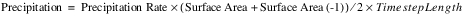where:
– Evaporation Coefficient = the Evaporation Coefficient for the current month
– Surface Area = the current Surface Area of the Reservoir
– Surface Area(-1) = the Surface Area of the Reservoir at the previous timestepPan and Ice Evaporation
The Pan and Ice Evaporation method is used to calculate the volume of evaporation with one of two methods based on the value of the Pan Ice Switch slot for each timestep. The Pan Ice Switch slot is used as an indicator of whether ice is present on the surface of the reservoir. A value of 1.0 in the Pan Ice Switch slot indicates that there is ice cover on the Reservoir that must be taken into account when Evaporation is calculated. A value of 0.0 or any number other than 1.0 in the Pan Ice Switch slot indicates that there is no ice on the surface of Reservoir. The following slots are those specifically associated with this method.
Slots Specific to This Method
Evaporation
Type: Series
Units: VOLUME
Description: Volume of water lost to evaporation during the current timestep
Information:
I/O: Output only
Elevation Area Table
Type: Table
Units: LENGTH vs AREA
Description: Pool Elevation vs Surface Area
Information:
I/O: Required input
K Factor
Type: Series Slot with Periodic Input
Units: VELOCITY per TEMPERATURE_F
Description: Factor relating average temperature, in degrees Fahrenheit, to evaporation rate
Information: This slot is a series slot, but the data can be input as a periodic relationship.
I/O: Optional but is required Input if the Pan Ice Switch slot is 1.0
Max Air Temperature
Type: Series
Units: TEMPERATURE in FARENHEIT
Description: Maximum air temperature during the timestep
Information:
I/O: Optional; required only if the Pan Ice Switch slot is 1.0
Min Air Temperature
Type: Series
Units: TEMPERATURE in FARENHEIT
Description: Minimum air temperature during the timestep
Information:
I/O: Optional; required if the Pan Ice Switch slot is 1.0
Pan Evaporation
Type: Series
Units: LENGTH per TIME
Description: Evaporation rate from the surface
Information:
I/O: Optional; only required if the Pan Ice Switch is 0.0
Pan Evaporation Coefficient
Type: Table
Units: DECIMAL
Description: Weighing factor for pan evaporation rate
Information:
I/O: Optional; required if the Pan Ice Switch slot is 0.0
Pan Ice Switch
Type: Series
Units: No Units
Description: Indicator of surface ice coverage for each timestep; 1.0 = ice; any other number or 0.0 = no ice.
Information: This slot is a series slot, but the data can be input as a periodic relationship.
I/O: Required input
Precipitation Rate
Type: Series
Units: LENGTH per TIME
Description: Precipitation intensity for a given timestep
Information:
I/O: Optional; defaults to 0.0 if not specified by the user.
Precipitation Volume
Type: Series
Units: VOLUME
Description: Precipitation flow rate multiplied by the length of the timestep
Information: Used in the mass balance to solve for storage
I/O: Output only
Surface Area
Type: SeriesSlot
Units: AREA
Description: Reservoir Surface Area from the Elevation Area Table
Information:
I/O: Output only
Surface Ice Coverage
Type: SeriesSlot
Units: DECIMAL
Description: Fraction of the Surface Area which is covered by ice
Information:
I/O: Optional; only used if the Pan Ice Switch slot is 1.0. Defaults to 0.0 for any timestep not specified by the user.
Method Details
If the Pan Ice Switch slot is equal to 1.0, ice is present and the following calculation is performed to compute evaporation: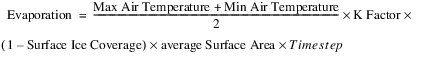If the calculated Evaporation is less than zero, the Evaporation is set equal to zero.
The Precipitation is calculated with the following equation if the Pan Ice Switch slot is equal to 1.0: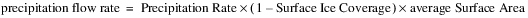The volume of precipitation that accumulated over the timestep at the Reservoir (Precipitation Volume) is the product of the precipitation flow rate and the timestep.
If the Pan Ice Switch slot is 0.0 or any number other than 1.0, there is no ice and the following calculation is performed to compute Evaporation: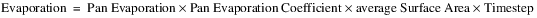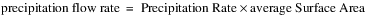The volume of precipitation that accumulated over the timestep at the Reservoir (Precipitation Volume) is the product of the precipitation flow rate and the timestep.Pan and Ice Evaporation, Current Surface Area
This method is exactly the same as the Pan and Ice Evaporation method. It uses the same slots, has the same required inputs and performs the same calculations. The only difference is that this method uses the instantaneous, end of timestep surface area instead of the average surface area over the timestep.Periodic Net Evaporation
Periodic Net Evaporation computes the gross evaporation from the reservoir and then subtracts out components of evaporation that would have occurred if the reservoir had not been built. This is the net evaporation and is set in the Evaporation slot. Each area of the submerged reservoir is separate including:
• River
• Streamside
• Terrace, and any
• Remaining areas
Each can have a separate evaporation coefficient and possibly additional components in its computation like temperature. The area of each region is specified in a separate table relating reservoir pool elevation to each region’s area. It is assumed that any precipitation that falls on the Remaining Area would have completely evaporated. The figure shows a sample of the different reservoir areas used in this method.
Figure 29.1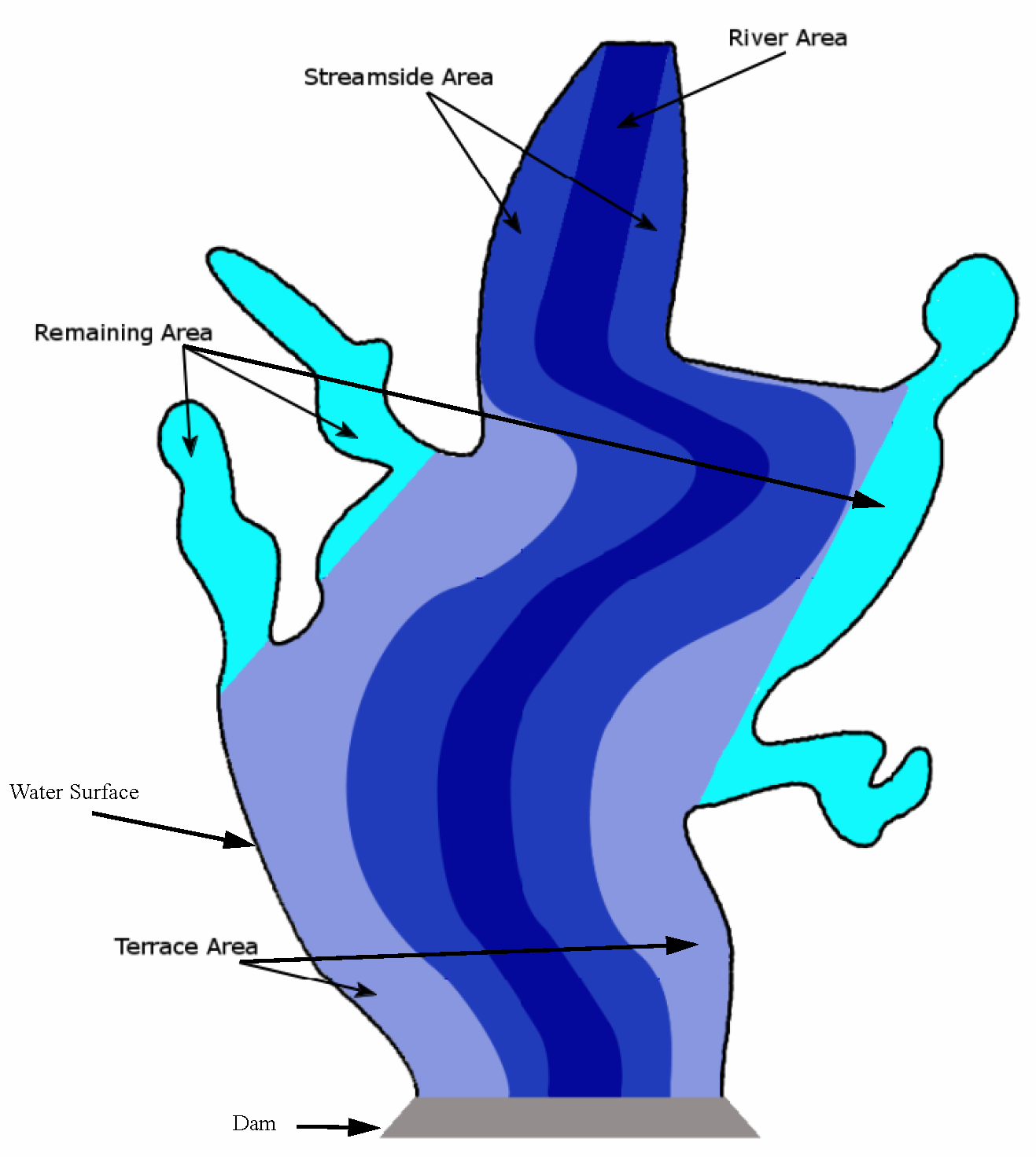Slots Specific to This Method
Average Precipitation
Type: Periodic
Units: Velocity (Length per Time)
Description: Slot describing the average precipitation
Information: Typically this would have a yearly period and monthly precipitation values. It is used in the computation of Remaining Evaporation.
I/O: Required input
Average Air Temperature
Type: Periodic
Units: TemperatureInFahren
Description: Slot describing the average air temperature
Information: Typically this would have a yearly period and monthly temperature values. It is used in computation of Streamside Evaporation and Terrace Evaporation.
I/O: Required input
Elevation Area Table
Type: Table
Units: Length vs Area
Description: Pool Elevation vs Surface Area
Information:
I/O: Required input
Elevation River Area
Type: Table
Units: Length vs Area
Description: Table relating reservoir Pool Elevation to submerged river area.
Information:
I/O: Required input
Elevation Streamside Area
Type: Table
Units: Length vs Area
Description: Table relating reservoir Pool Elevation to submerged streamside area.
Information:
I/O: Required input
Elevation Terrace Area
Type: Table
Units: Length vs Area
Description: Table relating Pool Elevation to submerged terrace area
Information:
I/O: Required input
Evaporation
Type: Series
Units: Volume
Description: Water lost from the reservoir to evaporation. This is the net evaporation and is the value that is included in the reservoir mass balance.
Information: This is the calculated as Gross Evaporation minus Salvage Evaporation
I/O: Output only
Gross Evaporation
Type: Series
Units: Volume
Description: The total evaporation off the reservoir surface. This is the evaporation that is actually occurring from the reservoir.
Information: This is calculated as GrossEvaporationCoeff times SurfaceAreaAvg converted from a flow to volume.
I/O: Output only
Gross Evaporation Coefficient
Type: Periodic
Units: Velocity (Length per Time)
Description: A table that describes the gross evaporation coefficient as it varies periodically. This is similar to a pan evaporation coefficient.
Information:
I/O: Required input
River Evaporation Coefficient
Type: Periodic
Units: Velocity (Length per Time)
Description: A table that describes the river evaporation coefficient as it varies periodically. This is similar to a pan evaporation coefficient.
Information:
I/O: Required input
Salvage Evaporation
Type: Series
Units: Volume
Description: The evaporation that would have occurred if the reservoir were not in place.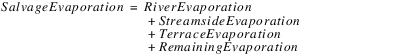Information:
I/O: Output only
Streamside Coefficient
Type: Periodic
Units: VelocityPerTemperature_F (that is, Length per Time per Temperature_F)
Description: Periodic table of coefficients
Information: Typically this represents a unit depth per month per degree Fahrenheit (for example, inches per month per degree Fahrenheit)
I/O: Required input
Surface Area
Type: Series
Units: Area
Description: Reservoir surface area computed from a lookup on the Elevation Area table
Information:
I/O: Output only
Terrace Coefficient
Type: Periodic
Units: VelocityPerTemperature_F (that is, Length per Time per Temperature_F)
Description: Periodic table of coefficients
Information: Typically this represents a unit depth per month per degree Fahrenheit (for example, inches per month per degree Fahrenheit)
I/O: Required input
Method Details
The method will be passed in a current estimate of Surface Area and Average Surface Area. The latter is an average of the current estimate and previous timestep’s value. In this description, it is called SurfaceAreaAvg. Similarly, StreamsideAreaAvg, RiverAreaAvg, TerraceAreaAvg and RemainingAreaAvg are all averages of the current and previous values.
In the following steps, the FlowToVolume and VolumeToFlow notation indicates that the specified expression will be converted from a flow to a volume (or vice versa) using the timestep length. This is necessary for the units to work correctly as evaporation [Volume units] is computed as a coefficient [Length/Time units] times an area [L2 units].
Note:  That in the following steps, the slots are in bold while intermediate values are not.
The method does the following:
1. Get the value from the periodic Gross Evaporation Coefficient slot. If not valid, issue an error.
2. Compute Gross Evaporation: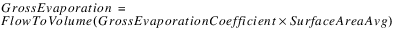3. Get the value from the periodic River Evaporation Coefficient slot. If not valid, issue an error.
4. Look up the Pool Elevation at t and t-1 on the Elevation River Area table to get the RiverArea at t and t-1. Then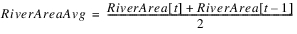5. Compute River Evaporation: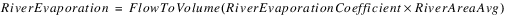This simulates that the river evaporation that would have occurred without the reservoir is a function of area and coefficient.
6. Get the value from the periodic Streamside Coefficient slot. If not valid, issue an error.
7. Look up the Pool Elevation at t and t-1 on the Elevation Streamside Area table to get the StreamsideArea at t and t-1. Then: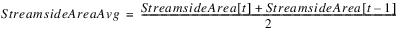8. Get the value from the periodic AverageTemperature slot. If not valid, issue an error.
9. Compute Streamside Evaporation: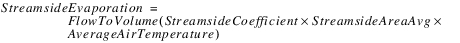This simulates that the streamside evaporation that would have occurred without the reservoir is a function of area, coefficient, and average air temperature.
10. Get the value from the periodic Terrace Coefficient slot. If not valid, issue an error.
11. Look up the Pool Elevation at t and t-1 on the Elevation Terrace Area table to get the Terrace Area at t and t-1. Then: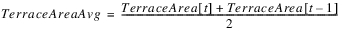12. Compute Terrace Evaporation: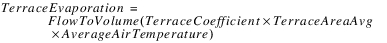This simulates that the terrace evaporation that would have occurred without the reservoir is a function of area, coefficient, and average air temperature.
13. Compute the average Remaining Area as follows: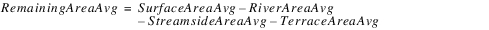If RemainingAreaAvg is less than zero, an error will be issued as the table data is incorrect.
14. Get the value from the periodic Average Precipitation. If not valid, issue an error.
15. Compute RemainingEvaporation: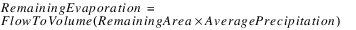This simulates that all of the precipitation on the Remaining Area would have evaporated.
16. Compute Salvage Evaporation:17. Compute Evaporation as follows: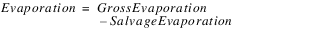The Evaporation is then a volume that is removed from the reservoir mass balance in the dispatch method.Single Evaporation
In the Single Evaporation method, evaporation is calculated linearly from the Single Evaporation Coefficient entered by the user. The total evaporated volume is a function of the average Reservoir Surface Area over the timestep, the Single Evaporation Coefficient, and the length of the timestep. The following slots are specifically associated with this method.
Slots Specific to This Method
Elevation Area Table
Type: Table Slot
Units: LENGTH vs AREA
Description: Pool Elevation vs Surface Area
Information:
I/O: Required input
Evaporation
Type: SeriesSlot
Units: VOLUME
Description: Volume of water lost to evaporation during one timestep
Information:
I/O: Output only
Single Evap Coeff
Type: Table Slot
Units: LENGTH PER time
Description: Rate of evaporation
Information: This slot contains a single value that represents the evaporation rate.
I/O: Required input
Precipitation Rate
Type: SeriesSlot
Units: LENGTH per TIME
Description: Precipitation intensity for the given timestep
Information: Value must be input by the user for each timestep.
I/O: Optional; defaults to 0.0 if not input.
Precipitation Volume
Type: SeriesSlot
Units: VOLUME
Description: Precipitation flow rate multiplied by the length of the timestep
Information: Used in the mass balance to solve for storage
I/O: Output only
Surface Area
Type: SeriesSlot
Units: AREA
Description: Reservoir Surface Area calculated from the Elevation Area Table
Information:
I/O: Output only
Method Details
The Surface Area of the Reservoir is calculated based on the Elevation Area Table. The Evaporation is then calculated using the following formula: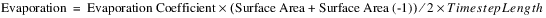The volume of Precipitation that occurred over the timestep is then calculated using the following equation:where:
– Evaporation Coefficient = SingleEvapCoeff entered by the user
– Surface Area = the current Surface Area of the Reservoir
– Surface Area(-1) = the Surface Area of the Reservoir at the previous timestep
Revised: 01/11/2023